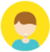Practical/Laboratory Manual Physics Class XI based on NCERT guidelines by Dr. J. P. Goel & Er. Meera Goyal - SBPD Publications

## NCERT Practical/Laboratory Manual Physics Class 11

Write a review 540 Views
An Excellent Book in Accordance with the latest syllabus for class 11 Prescribed by CBSE/NCERT and Adopted by Various State Education Boards.

Book Code : 0448/4864

ISBN : 978-93-5167-960-8

Language : Hindi

Edition : Latest

Availability : In Stock

Authors : Dr. J. P. Goel & Er. Meera Goyal

160.00 200.00 20% Off ( Coupon code applied successfully )
You will save : XXX after ** % Discount

Book Content

SECTION : A

EXPERIMENTS

1.Measurement of Length

1.To measure the diameter of a small spherical/cylindrical body by using a vernier callipers, 2. To measure the dimensions of a given regular body of known mass, using vernier callipers and hence find its density, 3. To measure the internal diameter and depth of a given cylindrical vessel (say calorimeter/beaker) by using vernier callipers and hence find its internal volume (i.e., capacity)

Viva-voce

2. Screw Gauge/Micrometer

4.To determine the diameter of a given wire using a screw gauge and find its volume, 5. To find the thickness of a given sheet with the help of screw gauge, 6.To measure the volume of an irregular lamina by using a screw gauge

Viva-voce

3. Spherometer

7.To measure the radius of curvature of a given spherical surface (convex lens) by using a spherometer

Viva-voce

4.Mass and Weight

8.To determine the mass of two different objects using a beam balance

Viva-voce

5.Parallelogram Law of Vectors

9.To find the weight of a given body using parallelogram law of vectors

Viva-voce

6.Simple Pendulum (Measurement of Time)

10.Using a simple pendulum, plot L–T and L–T2 graphs. Hence find the effective length of a second's pendulum, using appropriate graphs

Viva-voce

7. Friction

11.To study the relationship between force of limiting friction and normal reaction and to find the coefficient of friction between a block and a horizontal surface,

Viva-voce

8. Motion of a Body Along an Inclined Plane

12. To find the downward force along an inclined plane, acting on a roller due to  gravitational pull of the earth and study its relationship with the angle of inclination by plotting graph between force and sin

Viva-voce

SECTION : B

EXPERIMENTS

1.Elasticity

1.To determine the Young's modulus of elasticity of the material of the wire, using Searle's apparatus Viva-voce

2.Spring Constant

2.To find the spring constant of a helical spring by plotting load-extension graph

Viva-voce

3. Boyle's Gas Law

3.To study the variation in volume with pressure for a sample of air constant temperature by plotting graphs between P and V and between P and 1/V   18

Viva-voce

4. Surface Tension

4.To determine the surface tension of water by capillary rise method

Viva-voce

5.Viscosity

5.To determine the co-effective of viscosity of given liquid by measuring the terminal velocity of a given spherical body in it

Viva-voce

6.Newton's Law of Cooling

6.To study the relationship between temperature of a hot body and time by plotting a cooling curv

Viva-voce

7.Vibrations of Strings

7. To study the relation between frequency and length for a given wire under constant tension using a sonometer

Viva-voce

8.To study the relation between the length of a given wire and tension for constant frequency using sonometer

Viva-voce

8.Vibrations of Air Columns

9.To find the velocity of sound in air at room temperature using a resonance tube by two resonance position

Viva-voce

9.Specific Heat

10.To determine specific heat of a given solid by the method of mixture

11.To determine the specific heat of a given liquid by method of mixture

Viva-voce

SECTION : A

ACTIVITIES

1.To make a paper scale of given least count e.g., 0.2 cm, 0.5 cm and use it to measure the length of a given object.  2.To determine the mass of a given body using a metre scale and by applying principle of moments.

Viva-voce

3.To plot a graph for a given set of data using proper choice of scales and error bars.

Viva-voce

4.To measure the force of limiting friction for rolling of a roller on horizontal plane.

Viva-voce

5.To study the variation in the range of a jet of water with angle of projection.

Viva-voce

6.To study the conservation of energy of a ball rolling down on inclined plane (using a double inclined plane).

Viva-voce

7. To study dissipation of energy of a simple pendulum by plotting a graph between square of amplitude and time.

Viva-voce

SECTION : B

ACTIVITIES

1.To observe the change of the state and plot a cooling curve for molten wax.

Viva-voce

2.To observe and explain the effect of heating on a bimetallic strip.

Viva-voce

3.To note the change in level of liquid in a container on heating and interprect the observations.

Viva-voce

4.To study the effect of detergent in surface tension by observing capillary rise.

Viva-voce

5.To study the factors affecting the rate of loss of heat of a liquid.

Viva-voce

6.To study the effect of load on depression of a suitably clamped meter scale loaded (i) at itsend (ii) in the middle.

Viva-voce

7.To observe the decrease in pressure with the increase in velocity of the fluid.

Viva-voce

APPENDIX

Some Important Tables of Physical Constants

Log-Antilog and other Tables

Book Details
• Author/Authors : Dr. J. P. Goel & Er. Meera Goyal
• Class : 11
• Page No. : 160
• Year : 2023-24
Write a review

### Write a review

Review must be between max 1000 characters!

## Customer ReviewsXYZ

Excellent as described in the picture. Outstanding quality.